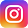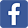# Stereogram: Smile by 3Dimka

[Back to Gallery] [Prev] 208 of 248 [Next]

```_~*(:=\$(+%_~*(:=\$(+%_~*(:=\$(+%_~*(:=\$(+%_~*(:=\$(+%
\$(+_~*:)= \$(+_~*:)= \$(+_~*:)= \$(+_~*:)= \$(+_~*:)=
(*)~/^=\$_ (*)~/^=\$_ (*)/^=\$_ (*)/^=\$:_ (*)/^=\$:_ (
[smile] :)[smile] :)smile] :)smile] :)s(mile] :)s(
O_o @LOL  O_o @LO  O_o @LO  O_o @LO  O_oi @LO  O_
_~*(:=\$(+%_~*(:=\$+%_~O*(:\$+%_~O*(:@\$+%~O*( :@\$+%~O
\$(+_~*:)= \$(+_~*)= \$(~+_~)= \$(~+_~:)= (~+_~::)= (~
(*)~/^=\$_ (*)~/=\$_ (*)~/=\$_ (*)~/=\$_ (*)~/=\$:_ (*)
[smile] :)[smil] :)[smil] :)[smil] :)[smil] \$:)[sm
O_o @LOL  O_o LOL  O_o LOL  O_o LOL  O_o LO L  O_
_~*(:=\$(+%_~*(:=(+%_ ~*(=(+%_ ~*(=(O+%_~*(=L(O+%_~
\$(+_~*:)= \$(+_~*:= \$(+*_~*:= \$(+*_~*: \$(+*(_~*: \$(
(*)~/^=\$_ (*)~/^=\$_(*)~/~^=\$_(*)~/^=\$_(*()~/^=\$_(*
[smile] :)[smile] :)smile] :)smile] :)s(mile] :)s(
O_o @LOL  O_o @LOL  O_ @LOL  O_ @LO L  O_ @LO L
_~*(:=\$(+%_~*(:=\$(+%_~*(:=\$(+%_~*(:=\$(+%_~*(:=\$(+%
by 3Dimka by 3Dimka by 3Dimka by 3Dimkaby 3Dimka b
_~*(:=\$(+%_~*(:=\$(+%_~*(:=\$(+%_~*(:=\$(+%_~*(:=\$(+%

```

*This stereogram is a courtesy of 3Dimka. You may not use this image without owner's permission.Add Comment: Hidden code: Your Name:Comments: Hidden Code: